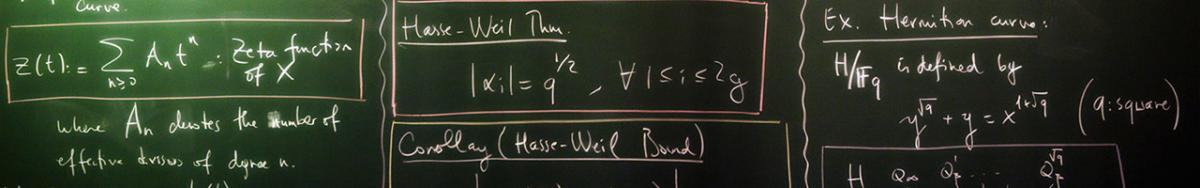•Mathematics

### About

Sabancı University has graduate programs leading to M.Sc and Ph.D degrees in Mathematics and a Minor Program in Mathematics aimed at students of engineering and economics programs. The research within the Mathematics Group at Sabancı University focus on:

• Analysis and its applications, differential equations (functional analysis, several complex variables, complex potential theory, holomorphic Dynamics, complex Geometry, probability and stochastics, martingales, operator theory, pseudo-differential operators, nonlinear partial differential equations).
• Algebra and number theory and their applications (finite fields and applications, coding theory, cryptology, integer partitions, combinatorics, commutative and computational algebra, finite geometry.